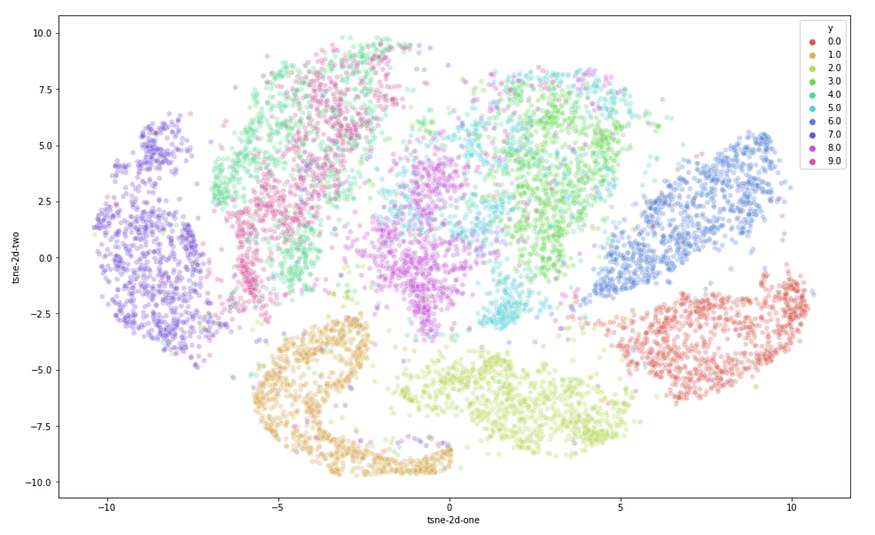Tags:

### Unsupervised Learning

• Outline

• Unsupervised learning
• Dimension reduction

• Principal component analysis (PCA)
• T-Distributed Stochastic Neighbor Embedding (t-SNE)
• Clustering

• K-means
• Hierarchical clustering

### Dimension Reduction

• Why dimension reduction ?

• compress data preserve useful information
• Data visulization

### Principlal Component analysis (PCA)

• PCA
• 把高維度的點，投影到低維度上面，且希望在低維度空間中保有在高維度中的性質！PCA in general

• Perform PCA by computing the eigenvectors of the k largest eigenvalues of the covariance matrix

PCA on MNIST### T-SNE (T-distributed Stochastic Neighbor Embedding)

• Goal: find locations in low dimensions such that the distance between points are preserved

• T-SNE allows non-linear transforms from the original data point to the new data point

T-SNEWhy different silmilarity measures?

• Crowding problem: distance between distant data points in the low dimension will not be large enough by SNE, which uses the same similarity measure for both low and high dimensional spaceT-SNE on MNISTSummary

• Both PCA and t-SNE project data points into low dimension

• PCA allows only linear projection
• T-SNE allows non-linear projection

• Interpretability
• Can project new data points

• Visualization

### Hierarchical clustering

• Types of hierarchical clustering

• Agglomerative (bottom-up)
• Merge two closest clusters until only one cluster left
• Divisive (top-down)
• Each step split a cluster until each cluster contains one data point

Agglomerateive exampleDifferent cluster resultSummary

• Clustering
• k-means and hierachical clustering

Tags:

Updated: